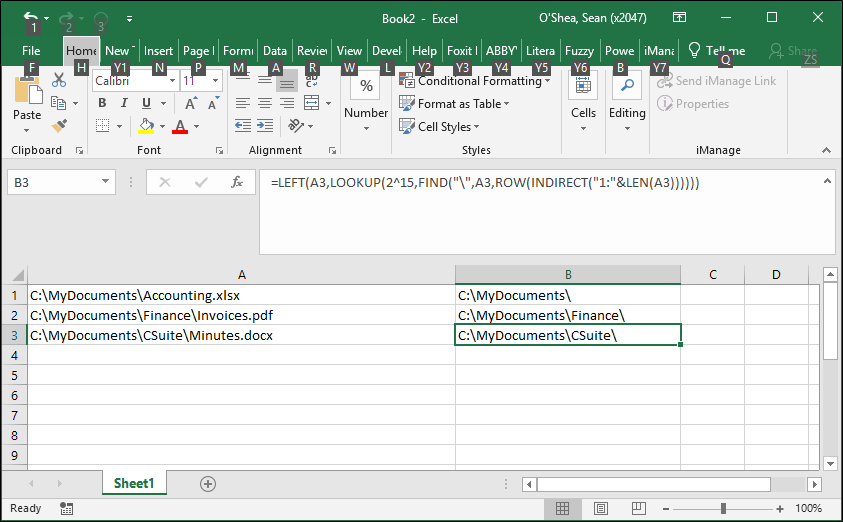Search

# Excel formula to extract folder path

The Excel formula posted here by barry houdini, allows you to pull the the folder path in a file path - the path absent the file name. So this formula:

=LEFT(A1,LOOKUP(2^15,FIND("\",A1,ROW(INDIRECT("1:"&LEN(A1))))))

. . . references the file path in cell A1.

For this file path:

C:\MyDocuments\CSuite\Minutes.docx

. . . the formula will pull the path 'C:\MyDocuments\CSuite'.